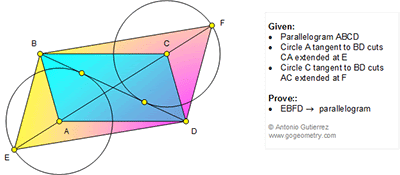## Friday, January 29, 2016

### Geometry Problem 1183: Parallelogram, Diagonals, Circle, Tangent Line

Geometry Problem. Post your solution in the comment box below.
Level: Mathematics Education, High School, Honors Geometry, College.

Click the figure below to view more details of problem 1183.1.Let AC meet BD at H
Observe that radius of circle A= radius of circle C
so AE=CF => HE=GF
H is the midpoint of EF and BD => EBFD is a parallelogram

2.tr ABD congr tr CBD =>altitudes A to tg point congr to C to tg point
=> Ra=Rb => EA=CF =>BD and EF halve each other